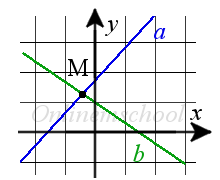Study of mathematics online.
Study math with us and make sure that "Mathematics is easy!"

# Online calculator. Point of lines intersection

This online calculator will help you to find the point of lines intersection.

Using this online calculator, you will receive a detailed step-by-step solution to your problem, which will help you understand the algorithm how to find point of two lines intersection.

## Find the point of two lines intersectionEquation of the 1st line:

y = x +

Equation of the 2nd line:

y = x +

You can input only integer numbers, decimals or fractions in this online calculator (-2.4, 5/7, ...). More in-depth information read at these rules.